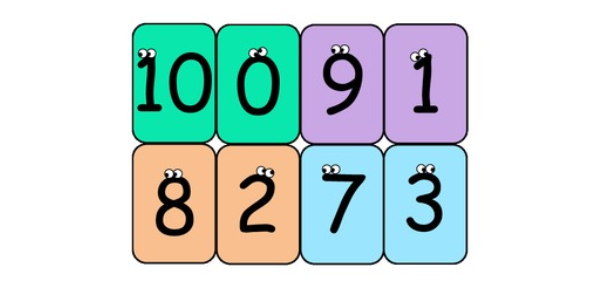# Mathematics For Kids- Addition Of Ten Quiz

20 Questions | Total Attempts: 42SettingsThis is a mathematics quiz for kids about adding ten to various numbers. It must not be difficult any how for any kid to pull this quiz out. So, let's swear on numbers and get started.

• 1.
1+10=
• 2.
9+10=
• 3.
10+6=
• 4.
8+10=
• 5.
10+9=
• 6.
10+3=
• 7.
7+10=
• 8.
4+10=
• 9.
2+10=
• 10.
10+10=
• 11.
10+7=
• 12.
10+2=
• 13.
6+10=
• 14.
10+4=
• 15.
10+5=
• 16.
10+1=
• 17.
3+10=
• 18.
10+10=
• 19.
5+10=
• 20.
10+8=
Related TopicsBack to top